Formulae based

Chapter 2 Class 12 Inverse Trigonometric Functions
Concept wise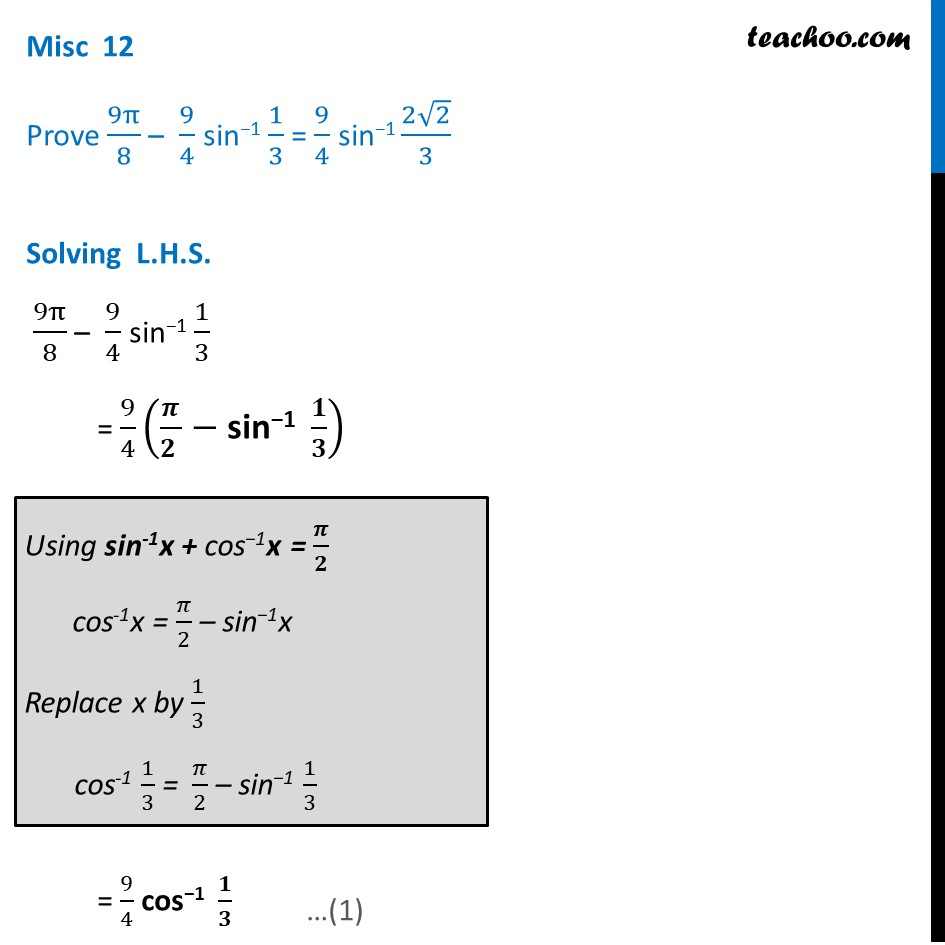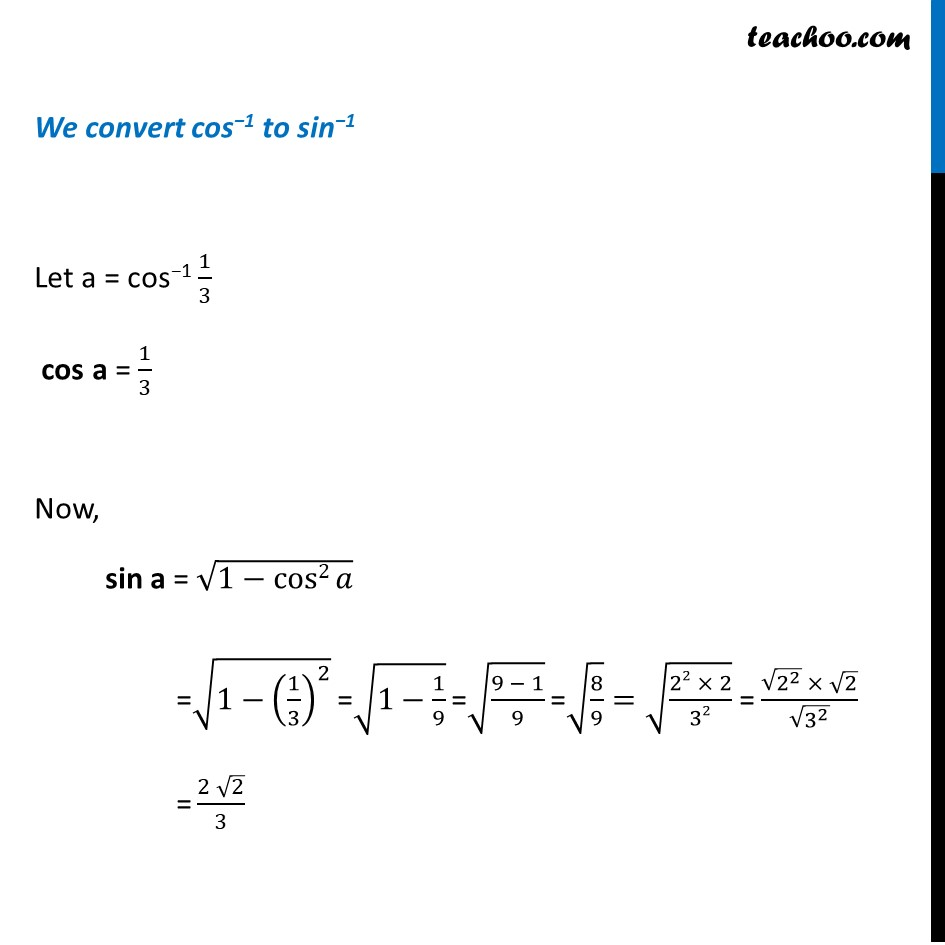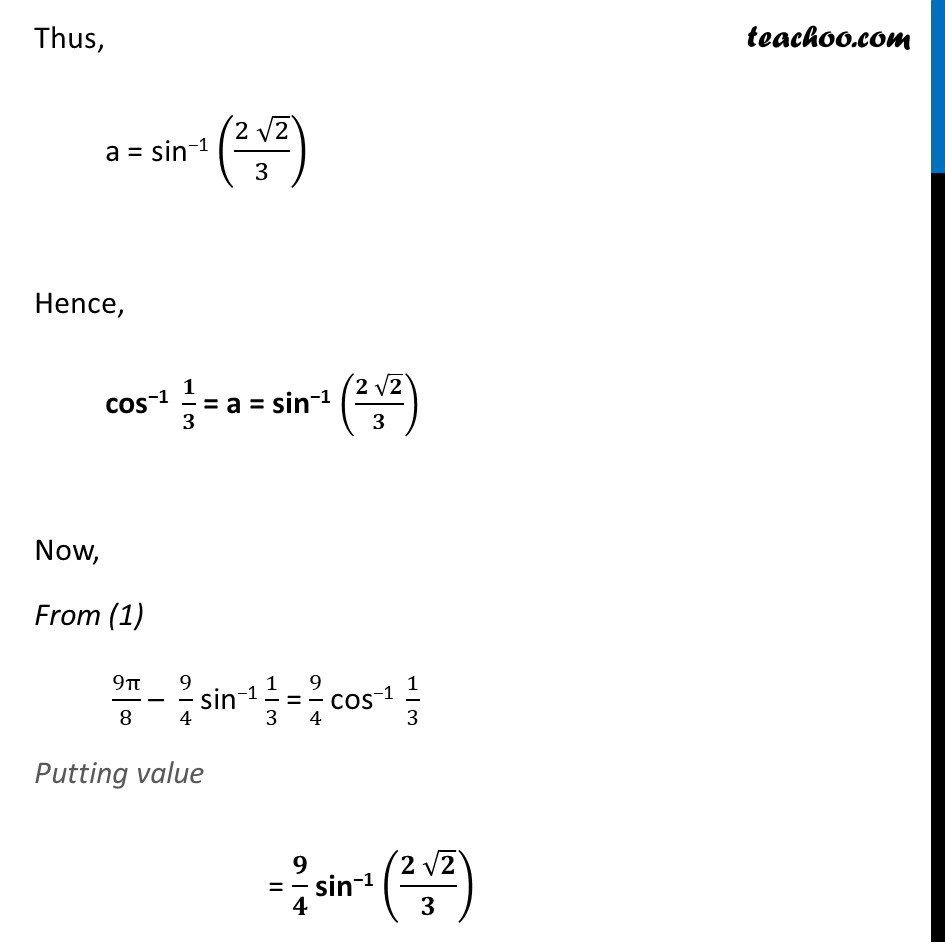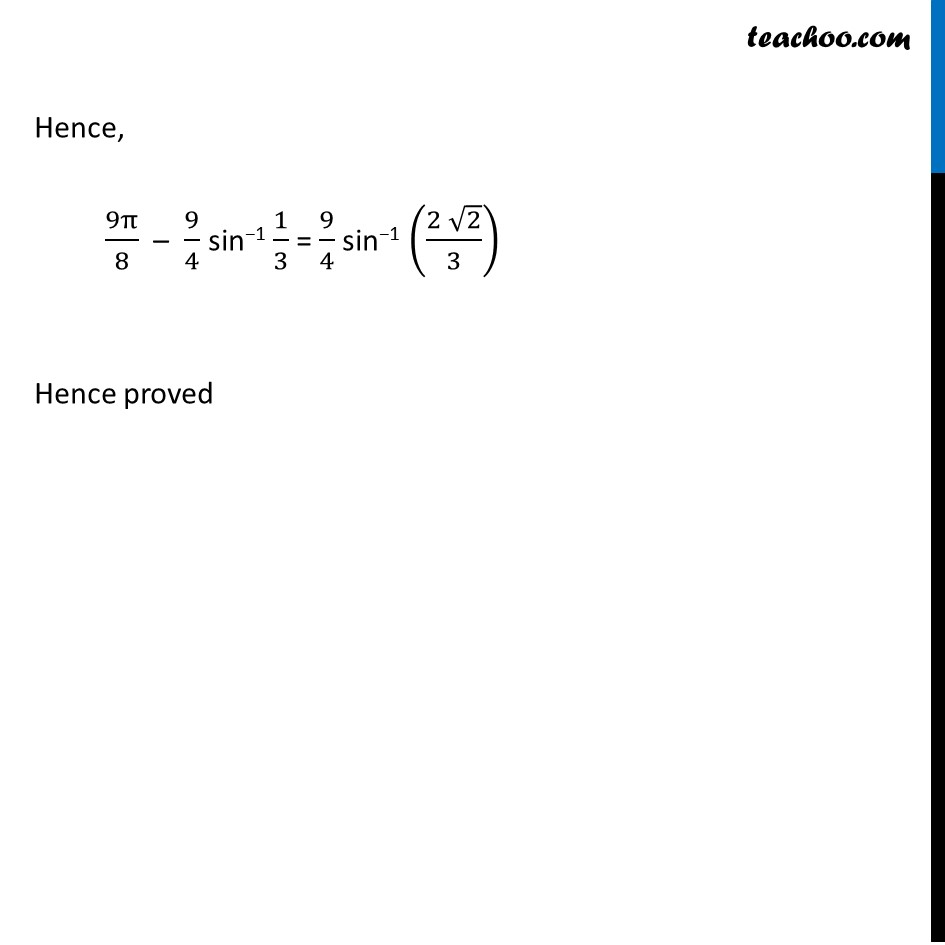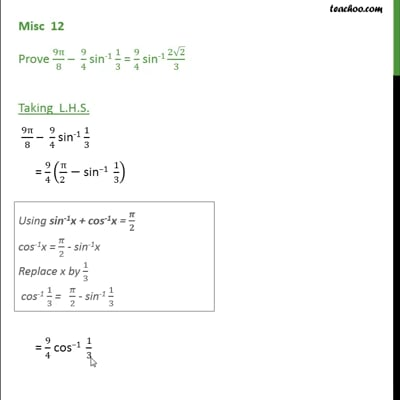This video is only available for Teachoo black users

Solve all your doubts with Teachoo Black (new monthly pack available now!)

### Transcript

Misc 12 Prove 9π/8 – 9/4 sin−1 1/3 = 9/4 sin−1 (2√2)/3 Solving L.H.S. 9π/8 – 9/4 sin−1 1/3 = 9/4 (𝝅/(𝟐 )−"sin−1 " 𝟏/𝟑) = 9/4 "cos−1 " 𝟏/𝟑 Using sin-1x + cos−1x = 𝝅/𝟐 cos-1x = 𝜋/2 – sin−1x Replace x by 1/3 cos-1 1/3 = 𝜋/2 – sin−1 1/3 We convert cos−1 to sin−1 Let a = "cos−1" 1/3 cos a = 1/3 Now, sin a = √(1−cos2 𝑎) =√(1−(1/3)^2 ) "=" √(1−1/9) "=" √((9 − 1)/9) "=" √(8/9)=√((22 × 2)/32) "=" (√(2^2 ) × √2)/√(3^2 ) "=" (2 √2)/3 Thus, a = sin−1 ((2 √2)/3) Hence, "cos−1 " 𝟏/𝟑 = a = sin−1 ((𝟐 √𝟐)/𝟑) Now, From (1) 9π/8 – 9/4 sin−1 1/3 = 9/4 "cos−1 " 1/3 Putting value = 𝟗/𝟒 sin−1 ((𝟐 √𝟐)/𝟑) Hence, 9π/8 – 9/4 sin−1 1/3 = 9/4 sin−1 ((2 √2)/3) Hence proved